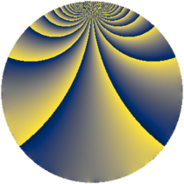# Properties

 Label 637.2.fLevel $637$ Weight $2$ Character orbit 637.f Rep. character $\chi_{637}(295,\cdot)$ Character field $\Q(\zeta_{3})$ Dimension $84$ Newform subspaces $12$ Sturm bound $130$ Trace bound $5$

# Related objects

## Defining parameters

 Level: $$N$$ $$=$$ $$637 = 7^{2} \cdot 13$$ Weight: $$k$$ $$=$$ $$2$$ Character orbit: $$[\chi]$$ $$=$$ 637.f (of order $$3$$ and degree $$2$$) Character conductor: $$\operatorname{cond}(\chi)$$ $$=$$ $$13$$ Character field: $$\Q(\zeta_{3})$$ Newform subspaces: $$12$$ Sturm bound: $$130$$ Trace bound: $$5$$ Distinguishing $$T_p$$: $$2$$, $$3$$, $$5$$

## Dimensions

The following table gives the dimensions of various subspaces of $$M_{2}(637, [\chi])$$.

Total New Old
Modular forms 148 104 44
Cusp forms 116 84 32
Eisenstein series 32 20 12

## Trace form

 $$84 q + 2 q^{2} - 34 q^{4} + 8 q^{5} - 6 q^{6} - 24 q^{8} - 26 q^{9} + O(q^{10})$$ $$84 q + 2 q^{2} - 34 q^{4} + 8 q^{5} - 6 q^{6} - 24 q^{8} - 26 q^{9} + 2 q^{10} - 2 q^{11} - 4 q^{12} + 2 q^{13} - 10 q^{15} - 10 q^{16} - 10 q^{17} - 20 q^{18} + 8 q^{19} + 10 q^{20} - 4 q^{22} + 6 q^{23} - 28 q^{24} + 60 q^{25} + 12 q^{26} + 36 q^{27} - 8 q^{29} + 24 q^{30} + 4 q^{31} + 6 q^{32} - 22 q^{33} + 8 q^{34} - 26 q^{36} - 30 q^{37} + 52 q^{38} - 12 q^{39} + 8 q^{40} - 16 q^{41} + 56 q^{44} + 10 q^{45} + 48 q^{46} - 28 q^{47} - 26 q^{48} + 24 q^{50} + 40 q^{51} - 78 q^{52} + 28 q^{53} - 6 q^{55} - 10 q^{58} + 10 q^{59} - 12 q^{60} - 24 q^{61} + 4 q^{62} - 72 q^{64} - 56 q^{65} - 12 q^{66} + 16 q^{67} - 66 q^{68} + 4 q^{69} - 14 q^{71} + 22 q^{72} + 16 q^{73} + 50 q^{74} - 14 q^{75} + 18 q^{76} + 110 q^{78} - 72 q^{79} + 34 q^{80} + 54 q^{81} - 28 q^{82} - 12 q^{83} + 42 q^{85} - 64 q^{86} - 10 q^{87} + 30 q^{88} + 32 q^{89} - 116 q^{90} - 36 q^{92} + 36 q^{93} + 52 q^{94} - 14 q^{95} + 212 q^{96} + 26 q^{97} - 96 q^{99} + O(q^{100})$$

## Decomposition of $$S_{2}^{\mathrm{new}}(637, [\chi])$$ into newform subspaces

Label Dim $A$ Field CM Traces $q$-expansion
$a_{2}$ $a_{3}$ $a_{5}$ $a_{7}$
637.2.f.a $2$ $5.086$ $$\Q(\sqrt{-3})$$ None $$-1$$ $$-3$$ $$-6$$ $$0$$ $$q+(-1+\zeta_{6})q^{2}+(-3+3\zeta_{6})q^{3}+\zeta_{6}q^{4}+\cdots$$
637.2.f.b $2$ $5.086$ $$\Q(\sqrt{-3})$$ None $$-1$$ $$3$$ $$6$$ $$0$$ $$q+(-1+\zeta_{6})q^{2}+(3-3\zeta_{6})q^{3}+\zeta_{6}q^{4}+\cdots$$
637.2.f.c $4$ $5.086$ $$\Q(\sqrt{-3}, \sqrt{5})$$ None $$-3$$ $$-3$$ $$-6$$ $$0$$ $$q+(-1-\beta _{1}-\beta _{3})q^{2}+(-1-\beta _{1}-\beta _{3})q^{3}+\cdots$$
637.2.f.d $4$ $5.086$ $$\Q(\zeta_{12})$$ None $$0$$ $$2$$ $$0$$ $$0$$ $$q-\zeta_{12}^{2}q^{2}+(\zeta_{12}+\zeta_{12}^{2})q^{3}+(-1+\cdots)q^{4}+\cdots$$
637.2.f.e $4$ $5.086$ $$\Q(\sqrt{2}, \sqrt{-3})$$ None $$2$$ $$0$$ $$-4$$ $$0$$ $$q+(1+\beta _{1}+\beta _{2})q^{2}-\beta _{1}q^{3}+(2\beta _{1}+\beta _{2}+\cdots)q^{4}+\cdots$$
637.2.f.f $4$ $5.086$ $$\Q(\sqrt{2}, \sqrt{-3})$$ None $$2$$ $$0$$ $$4$$ $$0$$ $$q+(1+\beta _{1}+\beta _{2})q^{2}+\beta _{1}q^{3}+(2\beta _{1}+\beta _{2}+\cdots)q^{4}+\cdots$$
637.2.f.g $8$ $5.086$ 8.0.$$\cdots$$.7 None $$-4$$ $$0$$ $$0$$ $$0$$ $$q+(-1+\beta _{2})q^{2}+\beta _{6}q^{3}+\beta _{2}q^{4}+(\beta _{1}+\cdots)q^{5}+\cdots$$
637.2.f.h $8$ $5.086$ 8.0.$$\cdots$$.6 None $$-2$$ $$0$$ $$0$$ $$0$$ $$q+(-1-\beta _{2}-\beta _{6})q^{2}+(-\beta _{3}+\beta _{7})q^{3}+\cdots$$
637.2.f.i $8$ $5.086$ $$\mathbb{Q}[x]/(x^{8} - \cdots)$$ None $$1$$ $$1$$ $$14$$ $$0$$ $$q+\beta _{1}q^{2}+\beta _{5}q^{3}+(-1+\beta _{1}+\beta _{2}+\cdots)q^{4}+\cdots$$
637.2.f.j $12$ $5.086$ $$\mathbb{Q}[x]/(x^{12} - \cdots)$$ None $$2$$ $$-1$$ $$2$$ $$0$$ $$q+(-\beta _{1}-\beta _{5}+\beta _{11})q^{2}-\beta _{11}q^{3}+\cdots$$
637.2.f.k $12$ $5.086$ $$\mathbb{Q}[x]/(x^{12} - \cdots)$$ None $$2$$ $$1$$ $$-2$$ $$0$$ $$q+(-\beta _{1}-\beta _{5}+\beta _{11})q^{2}+\beta _{11}q^{3}+\cdots$$
637.2.f.l $16$ $5.086$ $$\mathbb{Q}[x]/(x^{16} + \cdots)$$ None $$4$$ $$0$$ $$0$$ $$0$$ $$q+(1+\beta _{4}+\beta _{10})q^{2}+(\beta _{8}-\beta _{15})q^{3}+\cdots$$

## Decomposition of $$S_{2}^{\mathrm{old}}(637, [\chi])$$ into lower level spaces

$$S_{2}^{\mathrm{old}}(637, [\chi]) \cong$$ $$S_{2}^{\mathrm{new}}(91, [\chi])$$$$^{\oplus 2}$$